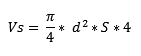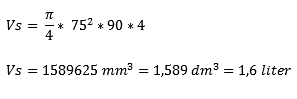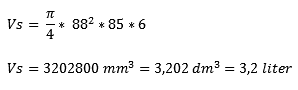# Bore and Stroke Volume

Subjects:

• Bore and Stroke Volume
• Calculate cylinder capacity
• Long stroke engine
• Square motor

Bore and Stroke Volume:
The cylinder capacity can be calculated with the bore and the stroke volume. The formula for a four-cylinder engine is as follows: Vs = π/4 x d² x S x 4

• Bore: This is the cylinder diameter, and is always shown in mm. (In the formula as d).
• Stroke: This is the distance the piston travels in the cylinder (from ODP to TDC). (In the formula as S).

This data is always stated in the data of a car manufacturer (eg a four-cylinder engine with the bore / stroke 75 X 90). This data can be calculated with a formula to a cylinder capacity.Calculating cylinder capacity:
The displacement of this four-cylinder engine is calculated with the formula:• We fill in the data of the engine (bore, stroke and number of cylinders). This results in an answer in cubic millimeters, which is converted to cubic decimeters. This is rounded off 1,6 litres.• Now let's take as an example a six-cylinder engine with a bore/stroke volume of 88/85. The cylinder capacity is 3,2 litres.Long stroke engine:
When the stroke length is greater than the bore, it is called a long stroke engine. (e.g. 75 X 90).

Square motor:
When the bore is greater than the stroke length, it is called a square engine. (e.g. 90 X 75).

Related pages:error: Alert: Content is protected !!